# The SURVEYFREQ Procedure

#### Wald Chi-Square Test

PROC SURVEYFREQ provides two Wald chi-square tests for independence of the row and column variables in a two-way table: a Wald chi-square test based on the difference between observed and expected weighted cell frequencies, and a Wald log-linear chi-square test based on the log odds ratios. These statistics test for independence of the row and column variables in two-way tables, taking into account the complex survey design. For information about Wald statistics and their applications to categorical data analysis, see Bedrick (1983); Koch, Freeman, and Freeman (1975); Wald (1943).

For these two tests, PROC SURVEYFREQ computes the generalized Wald chi-square statistic, the corresponding Wald F statistic, and also an adjusted Wald F statistic for tables larger than. Under the null hypothesis of independence, the Wald chi-square statistic approximately follows a chi-square distribution with (R – 1)(C – 1) degrees of freedom for large samples. However, it has been shown that this test can perform poorly in terms of actual significance level and power, especially for tables with a large number of cells or for samples with a relatively small number of clusters. See Thomas and Rao (1984, 1985) and Lohr (2010) for more information. See Felligi (1980) and Hidiroglou, Fuller, and Hickman (1980) for information about the adjusted Wald F statistic. Thomas and Rao (1984) found that the adjusted Wald F statistic provides a more stable test than the chi-square statistic, although its power can be low when the number of sample clusters is not large. See also Korn and Graubard (1990) and Thomas, Singh, and Roberts (1996).

If you specify the WCHISQ option in the TABLES statement, PROC SURVEYFREQ computes a Wald test for independence in the two-way table based on the differences between the observed (weighted) cell frequencies and the expected frequencies.

Under the null hypothesis of independence of the row and column variables, the expected cell frequencies are computed as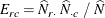where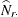is the estimated total for row r,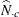is the estimated total for column c, and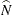is the estimated overall total, as described in the section Expected Weighted Frequency. The null hypothesis that the population weighted frequencies equal the expected frequencies can be expressed as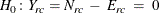for all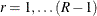and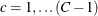. This null hypothesis can be stated equivalently in terms of cell proportions, with the expected cell proportions computed as the products of the marginal row and column proportions.

The generalized Wald chi-square statistic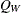is computed as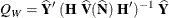where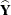is an array of (R – 1)(C – 1) differences between the observed and expected weighted frequencies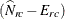, and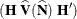estimates the variance of.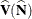is the covariance matrix of the estimates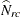, and its computation is described in the section Covariance of Totals.is an (R – 1)(C – 1) by RC matrix that contains the partial derivatives of the elements ofwith respect to the elements of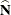. The elements ofare computed as follows, where a denotes a row different from row r, and b denotes a column different from column c: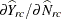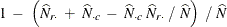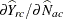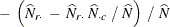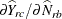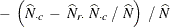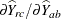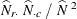Under the null hypothesis of independence, the statisticapproximately follows a chi-square distribution with (R – 1)(C – 1) degrees of freedom for large samples.

PROC SURVEYFREQ computes the Wald F statistic as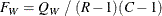Under the null hypothesis of independence,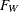approximately follows an F distribution with (R – 1)(C – 1) numerator degrees of freedom. The denominator degrees of freedom are the degrees of freedom for the variance estimator and depend on the sample design and the variance estimation method. The section Degrees of Freedom describes the computation of the denominator degrees of freedom. Alternatively, you can specify the denominator degrees of freedom with the DF= option in the TABLES statement.

For tables larger than, PROC SURVEYFREQ also computes the adjusted Wald F statistic as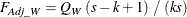where k = (R – 1)(C – 1), and s is the degrees of freedom, which are computed as described in the section Degrees of Freedom. Alternatively, you can specify the value of s with the DF= option in the TABLES statement. Note that fortables, k = (R – 1 )(C – 1) = 1, and therefore the adjusted Wald F statistic equals the (unadjusted) Wald F statistic, with the same numerator and denominator degrees of freedom.

Under the null hypothesis,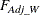approximately follows an F distribution with k numerator degrees of freedom and (sk + 1) denominator degrees of freedom.